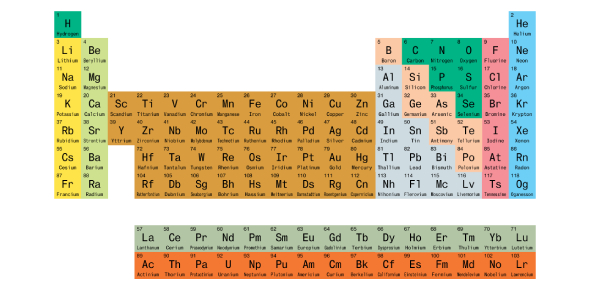# Periodic Table Of Elements: MCQ Quiz!

10 Questions | Total Attempts: 202Settings.

• 1.
The modern periodic table is organized by?
• A.

Size of atom

• B.

Atomic mass

• C.

Number of neutrons

• D.

Atomic number

• 2.
Elements in a group have:
• A.

A wide range of chemical properties

• B.

• C.

Similar chemical properties

• D.

The same number of protons

• 3.
Elements in a period:
• A.

Are horizontal rows on the periodic chart

• B.

Are vertical columns on the periodic chart

• C.

Have similar chemical properties

• D.

The same number of protons

• 4.
The elements in Group 1 of the periodic table are commonly called the
• A.

Alkali metals

• B.

Transition metals

• C.

Alkaline earth metals

• D.

Rare earth metals

• 5.
What does the number above the symbol for each element represent?
• A.

The number of isotopes

• B.

Its atomic number

• C.

Its number of neutrons

• D.

Its atomic mass

• 6.
The atom of what element is in Period 4, Group 13?
• A.

Na

• B.

Ga

• C.

Al

• D.

K

• 7.
What do the elements on the far right of the table (He, Ne, Ar, and Kr) have in common?
• A.

They do not generally react with other elements

• B.

They are liquid in normal conditions

• C.

They are metals that rust easily

• D.

They are very reactive gases

• 8.
Which of the following elements is in the same period as phosphorus?
• A.

Carbon

• B.

Magnesium

• C.

Nitrogen

• D.

Oxygen

• 9.
To what category of elements does an element belong if it is a poor conductor of electricity?
• A.

Transitional metals

• B.

Metalloids

• C.

Nonmetals

• D.

Metals

• 10.
Of the elements Pt, V, Li, and Kr, which is a nonmetal?
• A.

Pt

• B.

V

• C.

Li

• D.

Kr

Related TopicsBack to top